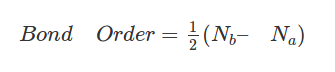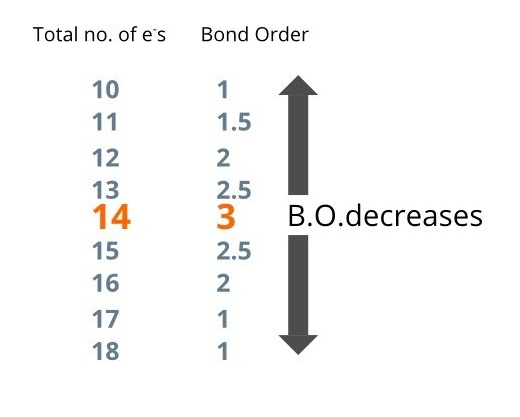# A quick and Handy tip to find the Bond Order in Chemical Bonding

Hello everyone,

I wanted to share this important concept of Bond order with all of you guys. One student asked me about the same. So, I thought to share it with everyone. It might be handy for you guys too.

Bond Order questions are very common in JEE and other entrance exams. So, I'll try to make it as simple as possible for you guys with my experience.

So, What is Bond Order?

Bond order is a number that tells us how strong the bond between two atoms forming a molecule is. If the bond order is high, the bond is strong and so the molecule is stable. If the bond order is low, the molecule is unstable.

How do we calculate the Bond Order?

There are many methods to finding bond order. One of the most famous and most used formulas states:where, Nb = No. of electrons in Bonding M.O.
and Na = No. of electrons in Anti-Bonding M.O.

Well, this is the formal method for finding bond order in questions but:

1.You need to properly find the number of electrons in bonding molecular orbitals and antibonding molecular orbitals.
2. And I think It takes a lot of time too.

So, How should you calculate bond order?

Just with some practice and memorization, you can find out the bond order of a species just by looking at it.

This method will work for any species that have between 10 and 18 electrons.
Most problems with respect to JEE have electrons between 10 and 18, therefore I think it is very handy.

1. First, what you need to remember is this: N2 has 14 electrons and its bond order is

So, Every electron added or subtracted to 14, reduces the bond order by 0.5.

For example, if the number of electrons is 15, then the bond order is 3 – 0.5 = 2.5. Done!

Look at the following table for more clarification:Steps:

1. Just count the total number of electrons and find the difference from 14. Call it ‘n’.
2. Bond order = 3 – 0.5n

Some Examples:

Q1. Find the bond order of CO.

Total number of electrons = 6 + 8 = 14. B.O. = 3.
Done! It’s that simple.

Q2. Find the B.O. of O22-

Total number of electrons = 8 x 2 + 2(for the – charge) = 18.

B.O. = 3 – 0.5 x 4 = 1

Q3. Which of the following has highest bond order?

``````a)O22+
b) O22-
c) F22-
d) O2+
``````

Just count the total number of electrons for each.

O22+ has 14 electrons. B.O = 3
O22- has 18 electrons. B.O = 1.

Same, You can find out the B.O. for the other two, and reached that the answer is (a).

Q4. Which of the following orders regarding the bond order is correct?

a) O2– > O2 > O2+
b) O2– < O2 < O2+
c) O2– > O2 < O2+
d) O2– < O2 >O2+

Once again, we count the number of electrons for each of these species.

For O2– no. of electrons = 17. B.O. = 1.5

For O2, no. of electrons = 16. B.O. = 2

For O2+, no. of electrons = 15. B.O. = 2.5

It's that simple.

Hope this method will reduce your effort and time also.

Thanks!

20 Likes

Thnx a lot sir. Outstanding trick. I founf it difficult to draw the mos in mind find the bo. It often led to mistakes. But now there is no problem!!!!!

1 Like

Waiting for more such tips bro..keep messaging me if u have more tips plz..

this saves hell lot of time man! cool

It is very helpful for me..